Courses

# SRMJEEE Chemistry Mock Test - 1

## 35 Questions MCQ Test SRMJEEE Subject Wise & Full Length Mock Tests | SRMJEEE Chemistry Mock Test - 1

Description
This mock test of SRMJEEE Chemistry Mock Test - 1 for JEE helps you for every JEE entrance exam. This contains 35 Multiple Choice Questions for JEE SRMJEEE Chemistry Mock Test - 1 (mcq) to study with solutions a complete question bank. The solved questions answers in this SRMJEEE Chemistry Mock Test - 1 quiz give you a good mix of easy questions and tough questions. JEE students definitely take this SRMJEEE Chemistry Mock Test - 1 exercise for a better result in the exam. You can find other SRMJEEE Chemistry Mock Test - 1 extra questions, long questions & short questions for JEE on EduRev as well by searching above.
QUESTION: 1

### Epichlorohydrin is

Solution: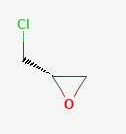QUESTION: 2

### Acetaldehyde and acetone differ in their reaction with

Solution: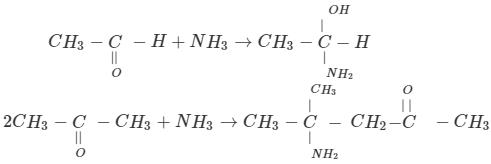As methyl group donates electrons, hydrogen atom withdraws electrons; this makes the molecule more polarized, and it makes the molecule more reactive. Compared to acetone, acetaldehyde has less stearic effects, and other molecules can approach easily. Due to these reasons, acetaldehyde is more reactive than acetone.

QUESTION: 3

### Among the following hydrocarbons which decolourise bromine water, but does not give precipitate with ammonical AgNO3 is

Solution:
QUESTION: 4

A triglyceride can have how many different acyl groups?

Solution: Esterification of glycerol will produce TG or PL. Glycerol with one acyl group is a monoacylglycerol (MAG), those with 2 acyl groups a diacylglycerol (DAG) and of course those with 3 a triacylglycerol or triglyceride molecule. There are very specific enzymes involved in each of the three esterification steps.
QUESTION: 5

Which of the following does not react with AgCl?

Solution: AgCl is insoluble in water and NaNO3 is soluble in water.and also the reverse reaction of the reaction u asked is highly stable and unidirectional.
QUESTION: 6

The order of filling of electrons in the orbitals of an atom is

Solution:
QUESTION: 7

In a mole of water vapours at STP, the volume actually occupied or taken by the molecules (i.e., Avogadro's No.xvolume of one molecule) is

Solution:
QUESTION: 8

Which of the following is aromatic?

Solution:
QUESTION: 9

Which of the following phenomenon will occur when two atoms of the elements having same spin of electron approach for bonding?

Solution:
QUESTION: 10

Pi-bonds do not alter the shape, but merely shorten the

Solution:

A carbon-carbon double bond consists of one sigma bond and one pi bond. This double bond is stronger than a single covalent bond (599 kJ/mol for C = C vs. 348 kJ/mol for C—C) and also shorter with an average bond length of 1.33 Angstroms (133 pm).

QUESTION: 11

Which of the following is the strongest acid?

Solution:
QUESTION: 12

Which relation is correct ?

Solution:
QUESTION: 13

Chemical equilibrium is dynamic in nature, because

Solution:

The two opposite processes, forward reaction and reverse reaction, keep on occurring in it all the time but at exactly equal rates (or equal speeds)
A chemical reaction does not stop after reaching the equilibrium state, only the rate of the forward and the reverse reactions become equal at the equilibrium state

QUESTION: 14

The order of reaction is decided by

Solution:
QUESTION: 15

The bond dissociation energies of H₂,Cl₂ and HCl are 104, 58 and 103 kcal respectively. The enthalpy of formation of HCl gas will be

Solution: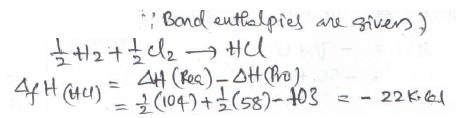QUESTION: 16

Soaps are classified as

Solution:
QUESTION: 17

The total number of acyclic isomers including stereoisomers of C₄H₇Cl are

Solution:

The total number of isomers, including the stereo-isomers, with formula C4H7Cl is 12. Out of this, 3 are cyclic and the remaining are acyclic.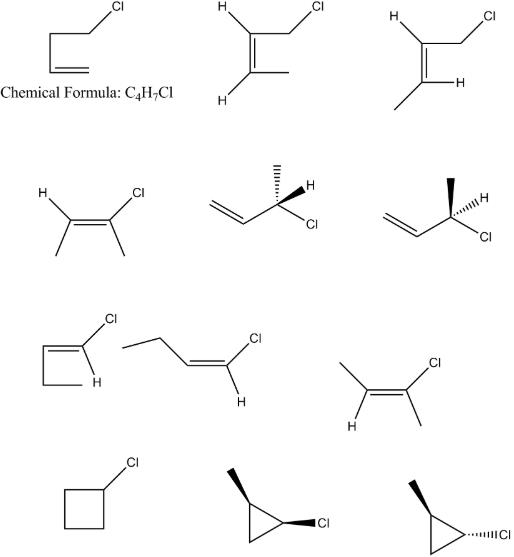QUESTION: 18

The charge required to liberate 112 ml of hydrogen from acidified water is

Solution: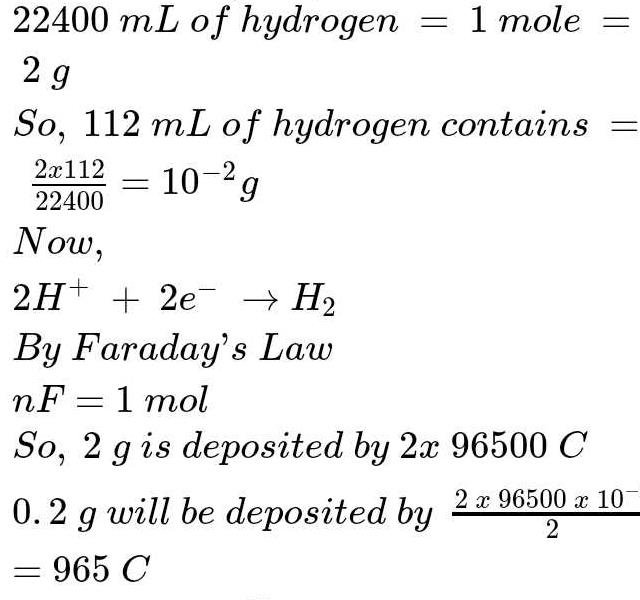QUESTION: 19

Which of the following ions are paramagnetic in character?

Solution:

Ni2+ is paramagnetic in nature as it has unpaired electrons.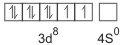QUESTION: 20

The value of gas constant per degree per mole is approximately

Solution: The value of gas constant per degree per mol is approximately 2 cal.

Note:
In the equation of state of an ideal gas Pv=nRT the value of universal gas constant would depend only on the units of measurement. It is independent of the nature of the gas, the pressure of the gas and the temperature of the gas.

For example:-R=8.314J/mol k

R=1.99 cal/mol /k
QUESTION: 21

When ammonium chloride is added to ammonia solution , the pH of the resulting solution will be

Solution: Ammonium chloride is a strong acid...ammonium hydroxide(solution) is weak base. So when ammonium chloride is added to ammonium solution,the pH of the solution goes from higher number to lower number..that is it decreases
QUESTION: 22

The alkene formed as a major product in the above elimination reaction is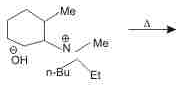Solution:
QUESTION: 23

A compound with molecular formula C₇H₁₆ shows optical isomerism, the compound will be

Solution:
QUESTION: 24

The phenomenon of radioactivity was discovered by

Solution:
QUESTION: 25

Calcium acetate on heating results in

Solution:
QUESTION: 26

Which of the following chemicals are used to manufacture methyl isocyanate that caused "Bhopal Tragedy " ? (a) Methyl amine (b) Phosgene (c) Phosphine (d) Dimethyl amine

Solution:
QUESTION: 27

Solution:
QUESTION: 28

Bakelite is a product formed from

Solution:
QUESTION: 29

If 0.183 of an aromatic monobasic acid requires 15ml of N/10 sodium hydroxide for complete neutralisation, then molecular weight of the acid is

Solution:

Milliequivalents of NaOH = 15×1/10 = 1.5 = 0.0015 equivalents
For neutralization equivalents of acid = 0.0015
As the acid is monobasic, n factor= 1, so moles of acid required = 0.0015/1 = 0.0015
weight/MW = 0.183g/0.0015 MW
MW= 122g

QUESTION: 30

What is oxidation number of Fe in Fe(CO)₅?

Solution: CO group is neutral. It has no charge due to this the charge on Fe is also zero.
QUESTION: 31

The second order Bragg diffraction of X-rays with λ = 1.00 Å from a set of parallel planes in a metal occurs at an angle of 60°. The distance between the scattering planes in the crystal is

Solution:
QUESTION: 32

If 20 ml of 0.4 N NaOH solution completely neutralises 40 ml of a dibasic acid, the molarity of the acid solution is

Solution: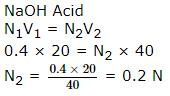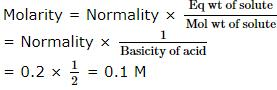QUESTION: 33

The vapour density of pure ozone would be

Solution:
QUESTION: 34

The maximum amount of BaSO₄ precipitated on mixing BaCl₂ (0.5M) with H₂SO₄ (1 M) will correspond to

Solution:
QUESTION: 35

Milk is an example of

Solution:

Milk is an example of the oil-in-water type of emulsion. In milk liquid fat globules are dispersed in water. Other examples are, vanishing cream etc.